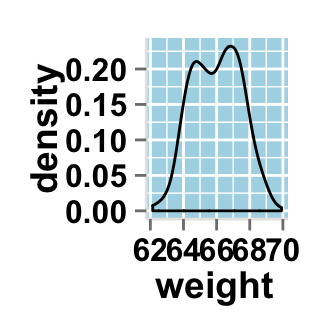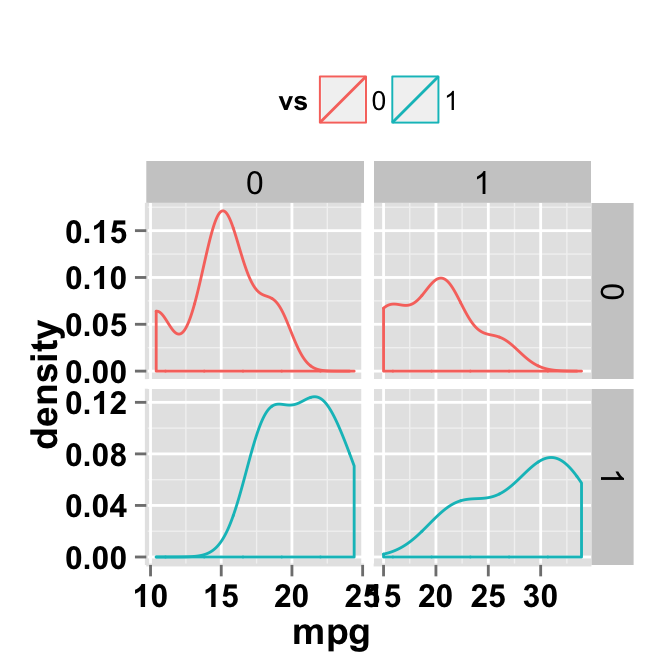# Introduction

ggplot2.density is an easy to use function for plotting density curve using ggplot2 package and R statistical software. The aim of this ggplot2 tutorial is to show you step by step, how to make and customize a density plot using ggplot2.density function. This function can also be used to personalize the different graphical parameters including main title, axis labels, legend, background and colors.

ggplot2.density function is from easyGgplot2 R package. An R script is available in the next section to install the package.

At the end of this tutorial you will be able to draw, with few R code, the following plots:ggplot2.density function is described in detail at the end of this document.

# Install and load easyGgplot2 package

easyGgplot2 R package can be installed as follow :

``````install.packages("devtools")
library(devtools)
install_github("easyGgplot2", "kassambara")``````

Load the package using this R code :

``library(easyGgplot2)``

# Data format

The data must be a numeric vector or a data.frame (columns are variables and rows are observations).

weight data, from easyGgplot2 package, will be used in the following examples.

``````# create a numeric vector
numVector<-rnorm(100)
``##  -0.8557 -0.6604  0.1977  2.0698 -1.1753 -0.5429``
``````# data.frame
``````##      sex weight
## 1 Female  63.79
## 2 Female  65.28
## 3 Female  66.08
## 4 Female  62.65
## 5 Female  65.43
## 6 Female  65.51``````

weight data contain the weight of 400 people (200 females and 200 males).

# Basic density plot

``````# Density of a single numeric vector
#ggplot2.density(data=numVector)
# Basic density plot from the vector "weight"
ggplot2.density(data=weight, xName='weight')
# Add mean line to the density plot
# Change the density line color, linetype and line size
ggplot2.density(data=weight, xName='weight',
meanLineType="dashed", meanLineSize=1,
colour="darkblue", linetype="dotted", size=1.5)
#Change the orientation: Horizontal density plot
ggplot2.density(data=weight, xName='weight',
orientation="horizontal")
# y Axis reversed
ggplot2.density(data=weight, xName='weight',
orientation="yAxisReversed")``````# Change the line type of the density plot

Different point shapes and line types can be used in the plot. By default, ggplot2 uses solid line type and circle shape.

``````# Change the density line color and line type
ggplot2.density(data=weight, xName='weight',linetype="longdash")``````# Density plot with multiple groups

``````# Multiple density curves on the same plot
# Color the density plot accoording to the groupName "grp"
ggplot2.density(data=weight, xName='weight', groupName='sex',
legendPosition="top")
# Density plots with semi-transparent fill.
# alpha is the transparency of the overlaid color
ggplot2.density(data=weight, xName='weight', groupName='sex',
legendPosition="top",
alpha=0.5, fillGroupDensity=TRUE )
# Density plots with mean lines
ggplot2.density(data=weight, xName='weight', groupName='sex',
legendPosition="top",# Parameters

The arguments that can be used to customize titles and x and y axis are listed below :

Parameters Description
mainTitle the title of the plot
mainTitleFont a vector of length 3 indicating respectively the size, the style (“italic”, “bold”, “bold.italic”) and the color of x and y axis titles. `Default value is: mainTitleFont=c(14, "bold", "black")`.
xShowTitle, yShowTitle if TRUE, x and y axis titles will be shown. Set the value to FALSE to hide axis labels. Default values are `TRUE`.
xtitle, ytitle x and y axis labels. Default values are `NULL`.
xtitleFont, ytitleFont a vector of length 3 indicating respectively the size, the style and the color of x and y axis titles. Possible values for the style:“plain”, “italic”, “bold”, “bold.italic”. Color can be specified as an hexadecimal code (e.g: “#FFCC00”) or by the name (e.g : “red”, “green”). Default values are `xtitleFont=c(14,"bold", "black"), ytitleFont=c(14,"bold", "black")`.
xlim, ylim limit for the x and y axis. Default values are `NULL`.
xScale, yScale x and y axis scales. Possible values : c(“none”, “log2”, “log10”). e.g: yScale=“log2”. Default values are `NULL`.
xShowTickLabel, yShowTickLabel if TRUE, x and y axis tick mark labels will be shown. Default values are `TRUE`.
xTickLabelFont, yTickLabelFont a vector of length 3 indicating respectively the size, the style and the color of x and y axis tick label fonts. Default value are `xTickLabelFont=c(12, "bold", "black"), yTickLabelFont=c(12, "bold", "black")`.
xtickLabelRotation, ytickLabelRotation Rotation angle of x and y axis tick labels. Default value are `0`.
hideAxisTicks if TRUE, x and y axis ticks are hidden. Default value is `FALSE`.
axisLine a vector of length 3 indicating respectively the size, the line type and the color of axis lines. Default value is `c(0.5, "solid", "#E5E5E5")`.

## Main title and axis labels

``````# Change main title and axis titles
ggplot2.density(data=weight, xName='weight',
mainTitle="Density curve",
xtitle="Weight (kg)", ytitle="Density")
# Customize title styles. Possible values for the font style :
# 'plain', 'italic', 'bold', 'bold.italic'.
ggplot2.density(data=weight, xName='weight',
xtitle="Weight (kg)", ytitle="Density",
mainTitle="Density curve",
mainTitleFont=c(14,"bold.italic", "red"),
xtitleFont=c(14,"bold", "#993333"),
ytitleFont=c(14,"bold", "#993333"))
# Hide x an y axis titles
ggplot2.density(data=weight, xName='weight',
xShowTitle=FALSE, yShowTitle=FALSE)``````### Axis ticks

``````# Axis ticks labels and orientaion
ggplot2.density(data=weight, xName='weight',
xShowTitle=FALSE, yShowTitle=FALSE,
xTickLabelFont=c(14,"bold", "#993333"),
yTickLabelFont=c(14,"bold", "#993333"),
xtickLabelRotation=45, ytickLabelRotation=45)
# Hide axis tick labels
ggplot2.density(data=weight, xName='weight',
xShowTitle=FALSE, yShowTitle=FALSE,
xShowTickLabel=FALSE, yShowTickLabel=FALSE)
# Hide axis ticks
ggplot2.density(data=weight, xName='weight',
xShowTitle=FALSE, yShowTitle=FALSE,
xShowTickLabel=FALSE,
yShowTickLabel=FALSE,hideAxisTicks=TRUE)
# AxisLine : a vector of length 3 indicating the size,
#the line type and the color of axis lines
ggplot2.density(data=weight, xName='weight',
axisLine=c(1, "solid", "darkblue"))``````## Background and colors

### Change density plot background and fill colors

``````# Change background color to "white". Default is "gray"
ggplot2.density(data=weight, xName='weight',
backgroundColor="white")
# Change background color to "lightblue" and grid color to "white"
ggplot2.density(data=weight, xName='weight',
backgroundColor="lightblue", gridColor="white")
# Change plot fill color
ggplot2.density(data=weight, xName='weight',
# Remove grid; remove top and right borders around the plot;
# change  axis lines
ggplot2.density(data=weight, xName='weight',
removePanelGrid=TRUE,removePanelBorder=TRUE,
axisLine=c(0.5, "solid", "black"))``````### Change density plot color according to the group

Colors can be specified as a hexadecimal RGB triplet, such as `"#FFCC00"` or by names (e.g : `"red"` ). You can also use other color scales, such as ones taken from the RColorBrewer package. The different color systems available in R have been described in detail here.

To change density plot color according to the group, you have to specify the name of the data column containing the groups using the argument `groupName`. Use the argument `groupColors`, to specify colors by `hexadecimal` code or by `name`. In this case, the length of groupColors should be the same as the number of the groups. Use the argument `brewerPalette`, to specify colors using `RColorBrewer`palette.

``````# Change group colors using hexadecimal colors
# alpha is the transparency level of overlaid color.
#The value can variate from 0 (total transparency)
#to 1 (no transparency)
ggplot2.density(data=weight, xName='weight', groupName='sex',
groupColors=c('#999999','#E69F00'),
fillGroupDensity=TRUE, alpha=0.5,
legendPosition="top")
# Change group colors using brewer palette: "Paired"
ggplot2.density(data=weight, xName='weight', groupName='sex',
brewerPalette="Paired",
fillGroupDensity=TRUE, alpha=0.5,
legendPosition="top")``````Color can also be changed by using names as follow :

``````# Change group colors using color names
ggplot2.density(data=weight, xName='weight', groupName='sex',
groupColors=c('aquamarine3','goldenrod1'),
fillGroupDensity=TRUE, alpha=0.5 )``````

## Legend

### Legend position

``````# Change the legend position to "top"
# (possible values: "left","top", "right", "bottom")
ggplot2.density(data=weight, xName='weight', groupName='sex',
legendPosition="top")
# legendPosition can be also a numeric vector c(x, y)
ggplot2.density(data=weight, xName='weight', groupName='sex',
legendPosition=c(0.8,0.2))
# Remove legend
ggplot2.density(data=weight, xName='weight', groupName='sex',
showLegend=FALSE)``````It is also possible to position the legend inside the plotting area. You have to indicate the x, y coordinates of legend box. x and y values must be between 0 and 1. `c(0,0)` corresponds to `"bottom left"` and `c(1,1)` corresponds to `"top right"` position.

### Legend background color, title and text font styles

``````# Change legend background color, title and text font styles
ggplot2.density(data=weight, xName='weight', groupName='sex',
# legendTitleFont=c(size, style, color)
legendTitle="Groups", legendTitleFont=c(10, "bold", "blue"),
#legendTextFont=c(size, style, color)
legendTextFont=c(10, "bold.italic", "red"),
#legendBackground: c(fill, lineSize, lineType, lineColor)
legendBackground=c("lightblue", 0.5, "solid", "darkblue" )
)``````## Axis scales

Possible values for x axis scale are “none”, “log2” and log10. Default value is “none”.

``````# Change x axis limit
ggplot2.density(data=weight, xName='weight', groupName='sex',
showLegend=FALSE, xlim=c(60,72))
# x Log scale. Possible values="none", "log2" and "log10"
ggplot2.density(data=weight, xName='weight', groupName='sex',
showLegend=FALSE, xScale="log2")``````## Create a customized plots with few R code

``````#Customized density
ggplot2.density(data=weight, xName='weight', groupName='sex',
groupColors=c('#999999','#E69F00'), showLegend=FALSE,
backgroundColor="white",
xtitle="Weight (kg)", ytitle="Density",
mainTitle="Weight density curve \nper sex",
)
#Remove grid; Remove Top and right border around the plot
#and fill each density curve according to the group
ggplot2.density(data=weight, xName='weight', groupName='sex',
groupColors=c('#999999','#E69F00'), showLegend=FALSE,
backgroundColor="white",
xtitle="Weight (kg)", ytitle="Density",
mainTitle="Weight density curve \nper sex",
removePanelGrid=TRUE,removePanelBorder=TRUE,
axisLine=c(0.5, "solid", "black"),
fillGroupDensity=TRUE, alpha=0.5)``````The argument alpha is used to specify the transparency of colors.

# Facet : split a plot into a matrix of pannels

The facet approach splits a plot into a matrix of panels. Each panel shows a different subset of the data.

## Facet with one variable

``````#Facet according to the sex variable
ggplot2.density(data=weight, xName='weight',
groupName='sex', legendPosition="top",
faceting=TRUE, facetingVarNames="sex")
# Change the direction. possible values are "vertical", horizontal".
# default is vertical.
ggplot2.density(data=weight, xName='weight',
groupName='sex', legendPosition="top",
faceting=TRUE, facetingVarNames="sex",
facetingDirection="horizontal") ``````## Faceting with two variables

The mtcars data is used in the following examples.

``````data(mtcars)
``````##                    mpg cyl disp  hp drat    wt  qsec vs am gear carb
## Mazda RX4         21.0   6  160 110 3.90 2.620 16.46  0  1    4    4
## Mazda RX4 Wag     21.0   6  160 110 3.90 2.875 17.02  0  1    4    4
## Datsun 710        22.8   4  108  93 3.85 2.320 18.61  1  1    4    1
## Hornet 4 Drive    21.4   6  258 110 3.08 3.215 19.44  1  0    3    1
## Hornet Sportabout 18.7   8  360 175 3.15 3.440 17.02  0  0    3    2
## Valiant           18.1   6  225 105 2.76 3.460 20.22  1  0    3    1``````

mtcars (Motor Trend Car Road Tests) comprises fuel consumption and 10 aspects of automobile design and performance for 32 automobiles.

``````#Facet by two variables: vs and am.
#Rows are vs and columns are am
ggplot2.density(data=mtcars, xName='mpg', groupName='vs',
legendPosition="top",
faceting=TRUE, facetingVarNames=c("vs", "am"))
# Facet by two variables: reverse the order of the 2 variables
#Rows are am and columns are vs
ggplot2.density(data=mtcars, xName='mpg', groupName='vs',
legendPosition="top",
faceting=TRUE, facetingVarNames=c("am", "vs"))``````## Facet scales

By default, all the panels have the same scale (`facetingScales="fixed"`). They can be made independent, by setting scales to `free`, `free_x`, or `free_y`.

``````# Facet with free scales
ggplot2.density(data=mtcars, xName='mpg', groupName='vs',
legendPosition="top",
faceting=TRUE, facetingVarNames=c("vs", "am"),
facetingScales="free")``````As you can see in the above plot, y axis have different scales in the different panels.

## Facet label apperance

``````# Change facet text font. Possible values for the font style:
#'plain', 'italic', 'bold', 'bold.italic'.
ggplot2.density(data=mtcars, xName='mpg', groupName='vs',
legendPosition="top",
faceting=TRUE, facetingVarNames=c("vs", "am"),
facetingFont=c(12, 'bold.italic', "red"))
# Change the apperance of the rectangle around facet label
ggplot2.density(data=mtcars, xName='mpg', groupName='vs',
legendPosition="top",
faceting=TRUE, facetingVarNames=c("vs", "am"),
facetingRect=list(background="white", lineType="solid",
lineColor="black", lineSize=1.5)
)``````# ggplot2.density function

## Description

Plot easily a density plot with R package easyGgplot2.

## usage

``````ggplot2.density(data, xName, groupName=NULL,
meanLineType="dashed", meanLineSize=1,
densityFill=NULL,fillGroupDensity=FALSE,
colorGroupDensityLine=FALSE,
groupColors=NULL, brewerPalette=NULL,faceting=FALSE,...)``````

## Arguments

Arguments Descriptions
data data.frame or a numeric vector. Columns are variables and rows are observations.
xName The name of column containing x variable. Default value is NULL.
groupName The name of column containing group variable. This variable is used to color plot according to the group.
addMeanLine If TRUE, the mean line is added on the plot for each group. Default value is FALSE.
meanLineColor, meanLineType, meanLineSize mean line color, type and size.
densityFill Fill color of density plot. This is only considered when groupName=NULL.
fillGroupDensity If TRUE, density curve of each group is filled. Default value is FALSE.
colorGroupDensityLine If TRUE, density curve line are colored. Default value is FALSE.
groupColors Color of groups. groupColors should have the same length as groups.
brewerPalette This can be also used to indicate group colors. In this case the parameter groupColors should be NULL. e.g: brewerPalette=“Paired”.
…. Other arguments passed on to ggplot2.customize custom function or to geom_density functions from ggplot2 package.

The other arguments which can be used are described at this link : ggplot2 customize. They are used to customize the plot (axis, title, background, color, legend, ….) generated using ggplot2 or easyGgplot2 R package.

## Examples

``````library(easyGgplot2)
#plot
ggplot2.density(data=weight, xName='weight',groupName='sex',
groupColors=c('#999999','#E69F00'),
mainTitle="Density plot \nper sex",
xtitle="Weight (kg)", ytitle="Density")
#Or use this
plot<-ggplot2.density(data=weight, xName='weight',groupName='sex',
groupColors=c('#999999','#E69F00'))
plot<-ggplot2.customize(plot,
mainTitle="Density plot \nper sex",
xtitle="Weight (kg)", ytitle="Density")
print(plot)``````

# Easy ggplot2 ebook

Note that an eBook is available on easyGgplot2 package here.

September 2014 : First edition.

Licence : This document is under creative commons licence (http://creativecommons.org/licenses/by-nc-sa/3.0/).

# Infos

This analysis was performed using R (ver. 3.1.0), easyGgplot2 (ver 1.0.0) and ggplot2 (ver 1.0.0).